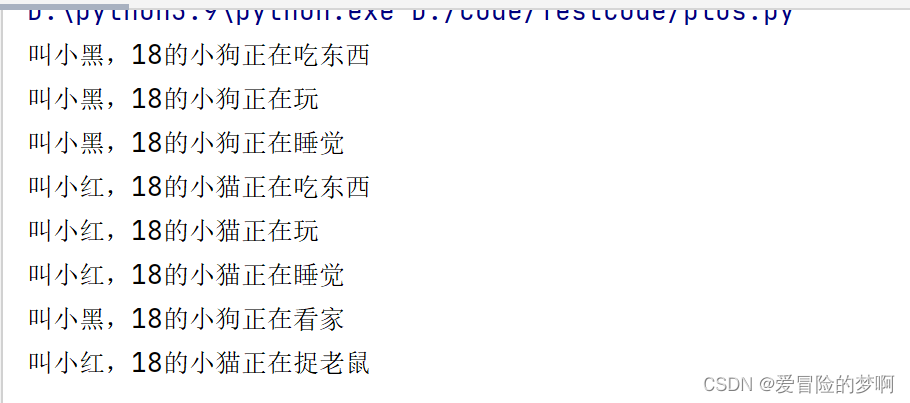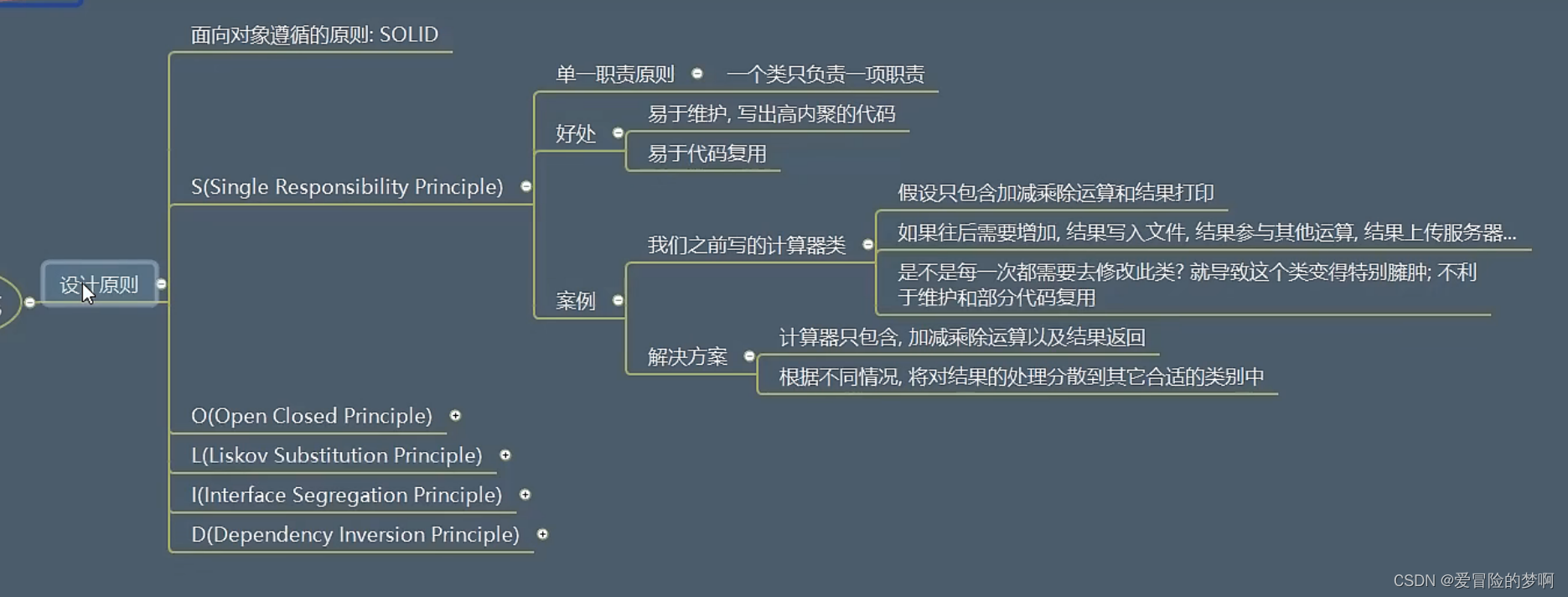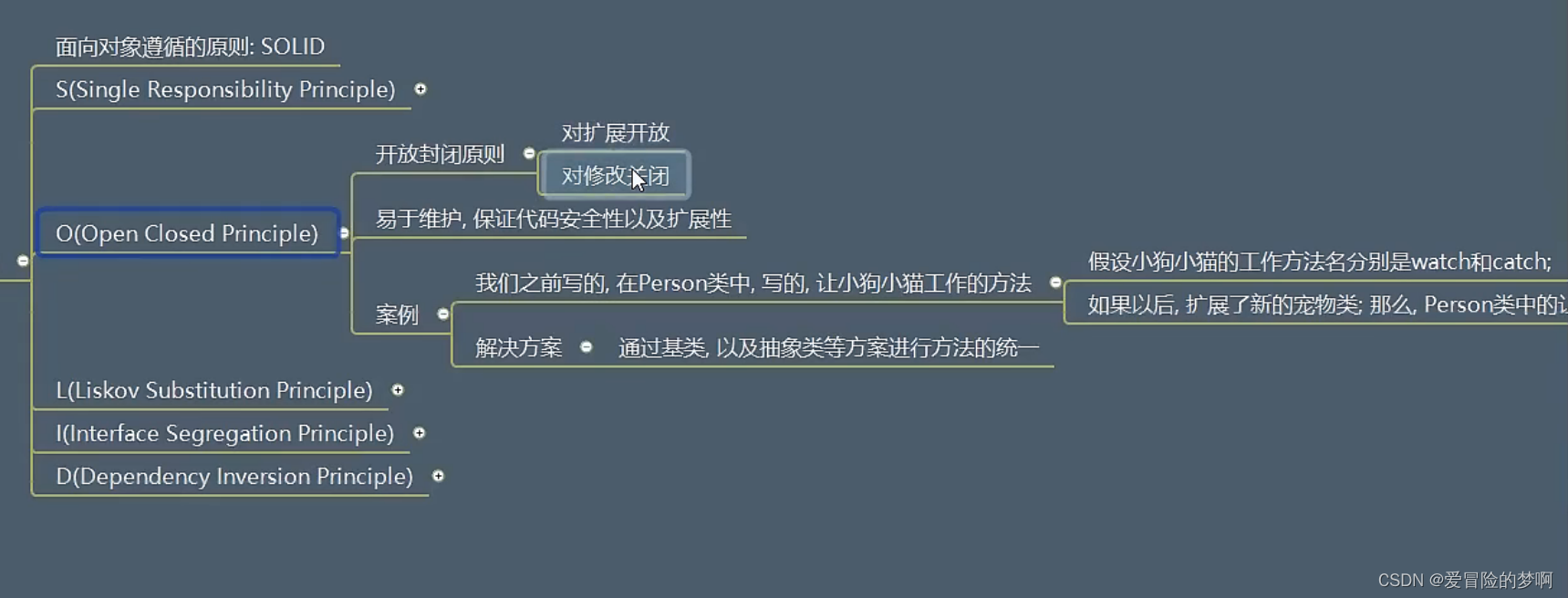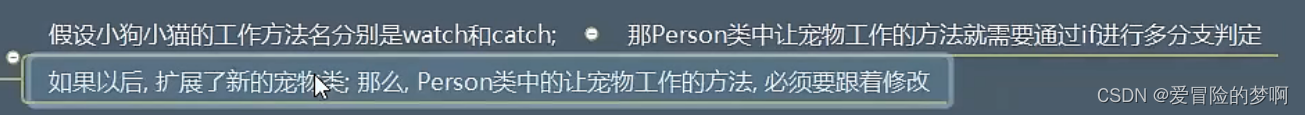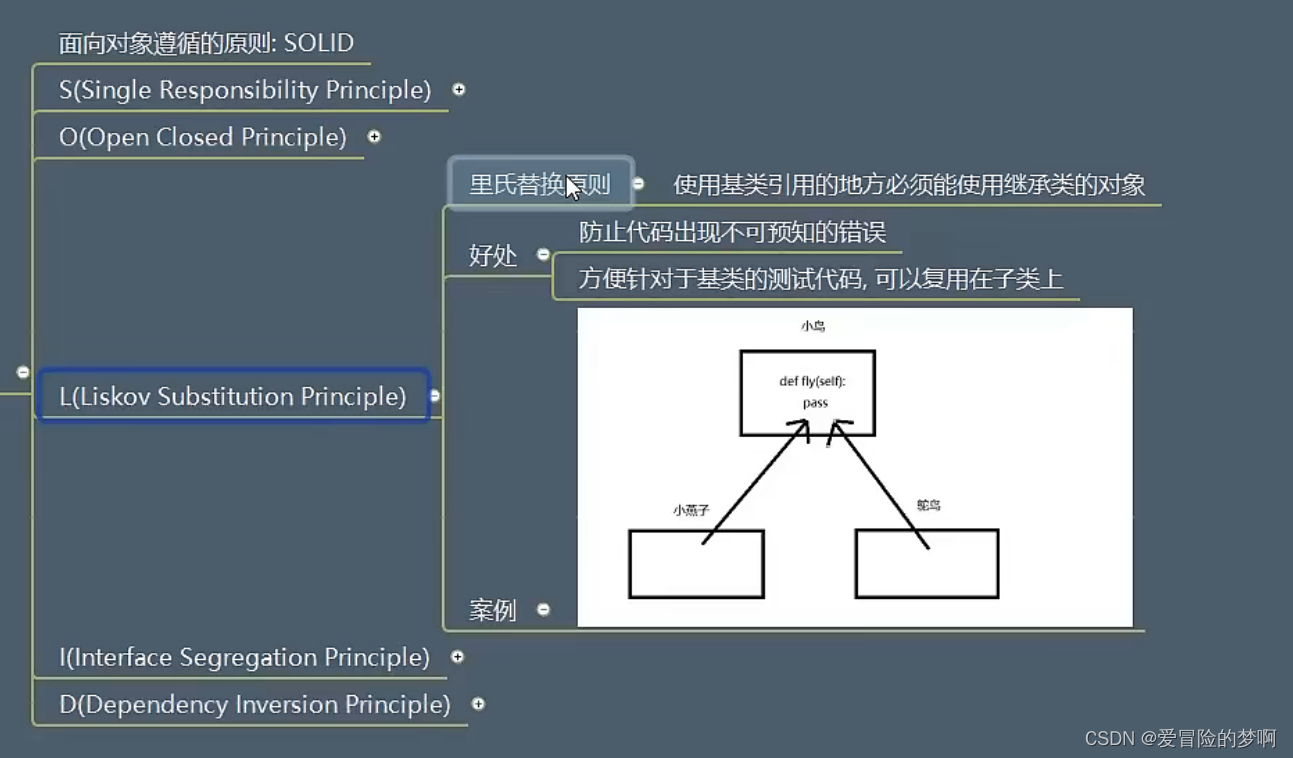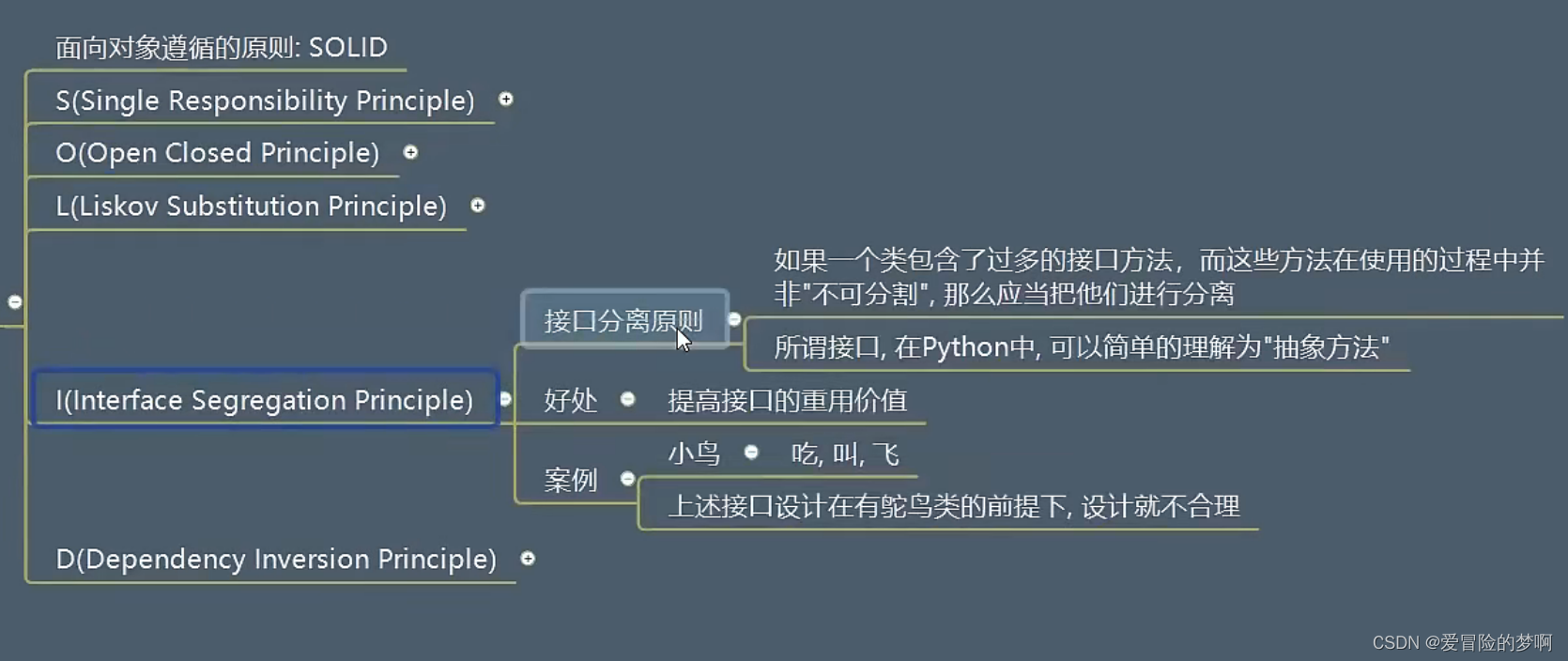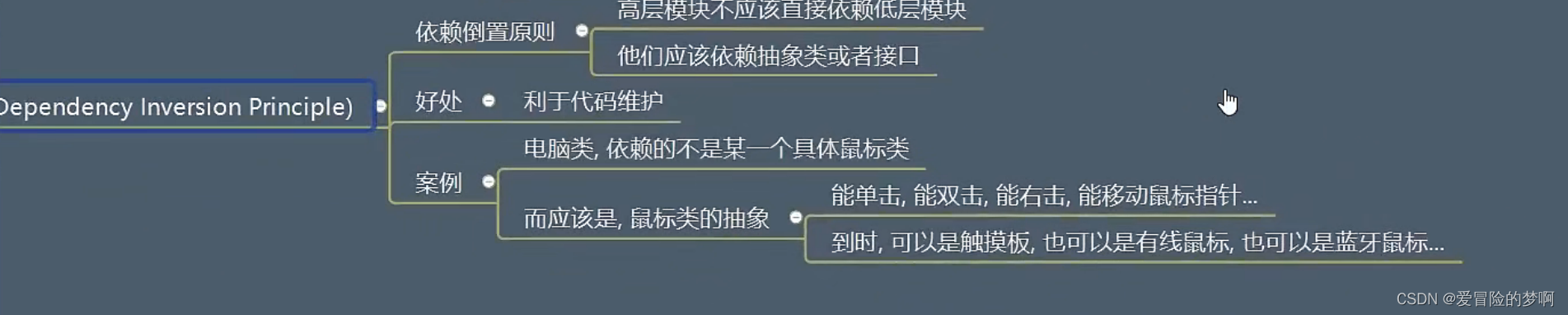# Python基础内容训练11（面向对象进阶）

## 面向对象进阶

1. 案例1 ：简单的计算器
``````# 实现一个计算器可以进行简单的基本操作，以及打印结果

def jia(n1, n2):
return n1 + n2

def jian(n1, n2):
return n1 - n2

def cheng(n1, n2):
return n1 * n2

a = jia(1, 2)
print(a)

#例如计算（6+2-4）*5
n1 = jia(6,2)
n2 = jian(n1,4)
n3 = cheng(n2,5)
print(n3)
``````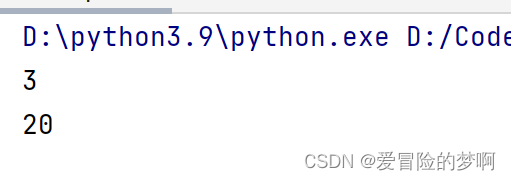• 改进1

``````result = 0
def first_value(v):
global result
result = v
def jia(n):
# 为了能让result可以在函数中修改,把他变成全局变量
global result
result += n
def jian(n):
global result
result -= n
def cheng(n):
global result
result *= n

# 计算（2+6-4）*5
first_value(2)
jia(6)
jian(4)
cheng(5)
print(result)
``````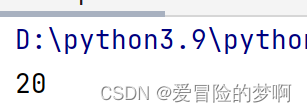• 改进2

``````class Caculator():
__result = 0

@classmethod
def first_value(cls,v):
cls.__result = v

@classmethod
def jia(cls,n):
cls.__result += n

@classmethod
def jian(cls,n):
cls.__result -= n

@classmethod
def cheng(cls,n):
cls.__result *= n

@classmethod
def show(cls):
print(f'计算的结果是:{cls.__result}')

Caculator.first_value(2)
Caculator.jia(6)
Caculator.jian(4)
Caculator.cheng(5)
Caculator.show()
``````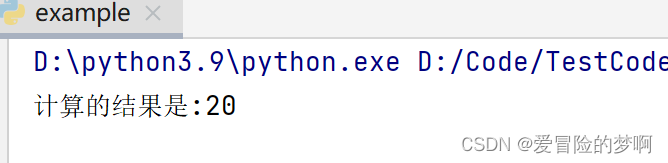• 改进3

``````class Caculator():

def __init__(self,result):
self.__result = result

def first_value(self,v):
self.__result = v

def jia(self,n):
self.__result += n

def jian(self,n):
self.__result -= n

def cheng(self,n):
self.__result *= n

def show(self):
print(f'计算的结果是:{self.__result}')

c1 = Caculator(2)
c1.jia(6)
c1.jian(4)
c1.cheng(5)
c1.show()
``````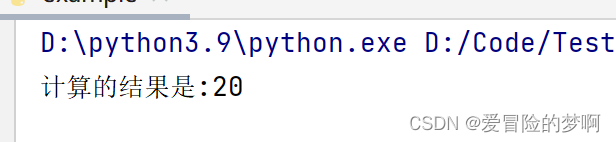• 改进4-加上验证、容错处理
``````class Caculator():
def check_num(self,num):
if not isinstance(num,int):
raise TypeError('您输入的类型错误')
def __init__(self,result):
self.check_num(result)
self.__result = result

def first_value(self,v):
self.check_num(v)
self.__result = v

def jia(self,n):
self.check_num(n)
self.__result += n

def jian(self,n):
self.check_num(n)
self.__result -= n

def cheng(self,n):
self.check_num(n)
self.__result *= n

def show(self):
print(f'计算的结果是:{self.__result}')

c1 = Caculator('abc')
c1.jia(6)
c1.jian(4)
c1.cheng(5)
c1.show()
``````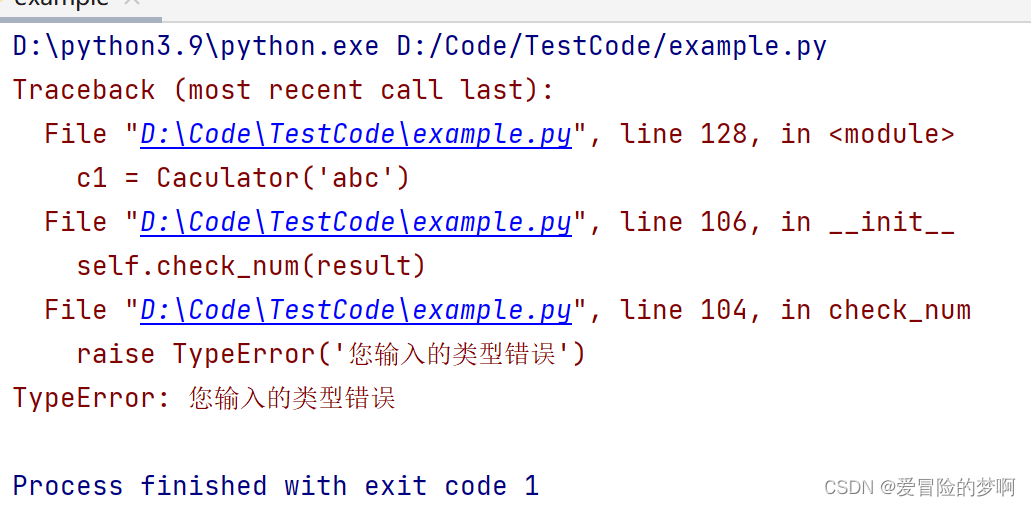• 改进5-上面的验证方法改变了代码原本的功能，我们可以使用装饰器来解决，可以加一些额外的功能，并且不会破坏原本的代码。

``````class Caculator():
#接受一个func，一个外界所传递过来的函数，也就是要装饰的函数
def check_num_zsq(func):
#内部呢，要定义一个闭包，要保证这个inner里面和下面加减乘所接受到的参数完全一样
def inner(self,n):
if not isinstance(n,int):
raise TypeError('您输入的类型错误')
#如果是整形，直接调用这个装饰的函数就可以了，并且把返回值返回给外界
return func(self,n)
#返回inner但是不要写小括号，小括号代表执行，不写小括号代表把函数给外界
return inner

@check_num_zsq
def __init__(self,result):
self.__result = result

@check_num_zsq
def first_value(self,v):
self.__result = v

@check_num_zsq
def jia(self,n):
self.__result += n

@check_num_zsq
def jian(self,n):
self.__result -= n

@check_num_zsq
def cheng(self,n):
self.__result *= n

def show(self):
print(f'计算的结果是:{self.__result}')

c1 = Caculator(2)
c1.jia(6)
c1.jian(4)
c1.cheng(5)
c1.show()
``````
• 改进6，已经写好了装饰器，装饰器本质讲就是一个函数，而这个函数写在类的内部是属于一个实例方法，外界可以通过实例来调用这个装饰器，但是没有什么意义，并且没什么用；那么怎么样不让外界调用这个装饰器呢？直接把它变成一个私有的方法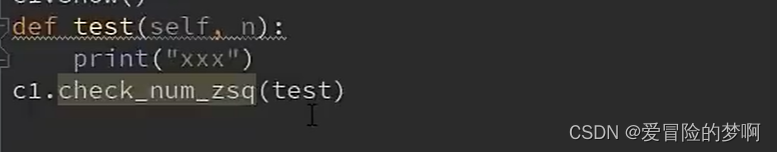``````class Caculator():
#接受一个func，一个外界所传递过来的函数，也就是要装饰的函数
def __check_num_zsq(func):
#内部呢，要定义一个闭包，要保证这个inner里面和下面加减乘所接受到的参数完全一样
def inner(self,n):
if not isinstance(n,int):
raise TypeError('您输入的类型错误')
#如果是整形，直接调用这个装饰的函数就可以了，并且把返回值返回给外界
return func(self,n)
#返回inner但是不要写小括号，小括号代表执行，不写小括号代表把函数给外界
return inner

@__check_num_zsq
def __init__(self,result):
self.__result = result

@__check_num_zsq
def first_value(self,v):
self.__result = v

@__check_num_zsq
def jia(self,n):
self.__result += n

@__check_num_zsq
def jian(self,n):
self.__result -= n

@__check_num_zsq
def cheng(self,n):
self.__result *= n

def show(self):
print(f'计算的结果是:{self.__result}')

c1 = Caculator(2)
c1.jia(6)
c1.jian(4)
c1.cheng(5)
c1.show()
``````
• 改进7-新增功能，输入的时候，加上语音播报，主要问题是这个语音播报写在那个位置，并且如何调用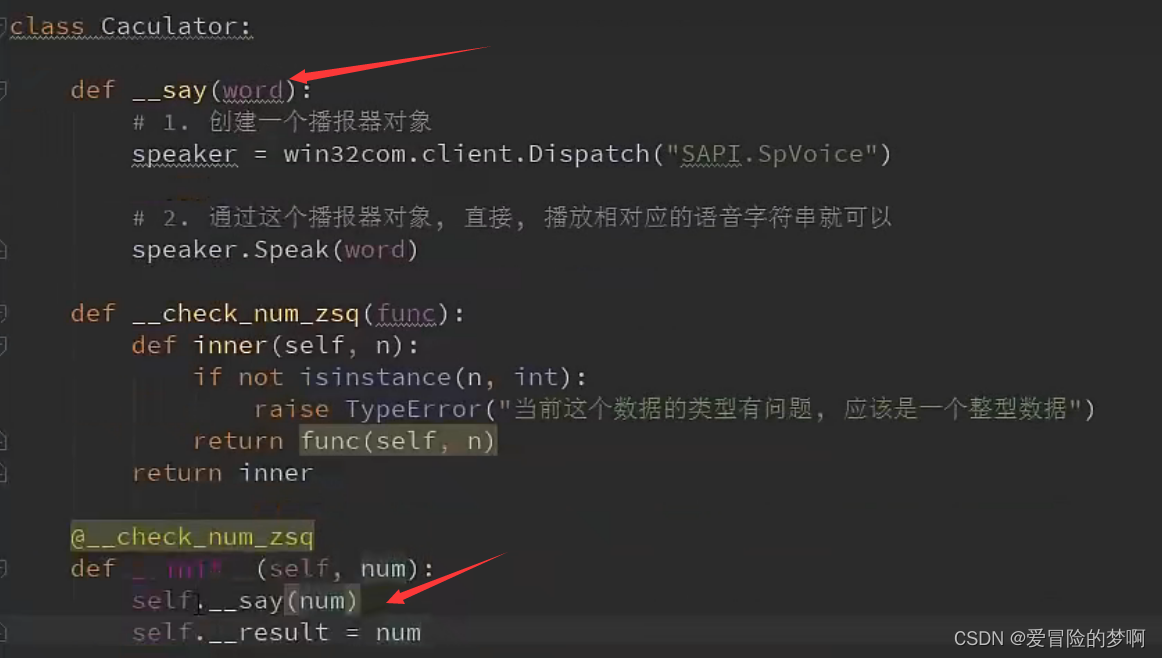``````python -m pip install pypiwin32
``````
``````import win32com.client
#1. 创建一个播报器对象
speaker = win32com.client.Dispatch('SAPI.SpVoice')
#2. 通过这个播报器对象，直接播放相对应的语音字符串即可
speaker.Speak('我的名字是sz')

class Caculator():
def __say(self,word):
# 1. 创建一个播报器对象
speaker = win32com.client.Dispatch('SAPI.SpVoice')
# 2. 通过这个播报器对象，直接播放相对应的语音字符串即可
speaker.Speak(word)
#接受一个func，一个外界所传递过来的函数，也就是要装饰的函数
def __check_num_zsq(func):
#内部呢，要定义一个闭包，要保证这个inner里面和下面加减乘所接受到的参数完全一样
def inner(self,n):
if not isinstance(n,int):
raise TypeError('您输入的类型错误')
#如果是整形，直接调用这个装饰的函数就可以了，并且把返回值返回给外界
return func(self,n)
#返回inner但是不要写小括号，小括号代表执行，不写小括号代表把函数给外界
return inner

@__check_num_zsq
def __init__(self,result):
self.__say(result)
self.__result = result

@__check_num_zsq
def first_value(self,v):
self.__say(v)
self.__result = v

@__check_num_zsq
def jia(self,n):
self.__say(n)
self.__result += n

@__check_num_zsq
def jian(self,n):
self.__say(n)
self.__result -= n

@__check_num_zsq
def cheng(self,n):
self.__say(n)
self.__result *= n

def show(self):
self.__say(f'计算的结果是:{self.__result}')
print(f'计算的结果是:{self.__result}')

c1 = Caculator(2)
c1.jia(6)
c1.jian(4)
c1.cheng(5)
c1.show()
``````
• 改进8-上面的代码还是一样的问题就是代码内容改了，所以为了保证代码的内容不被修改，我们继续可以使用装饰器来进行操作
``````class Caculator():

#接受一个func，一个外界所传递过来的函数，也就是要装饰的函数
def __check_num_zsq(func):
#内部呢，要定义一个闭包，要保证这个inner里面和下面加减乘所接受到的参数完全一样
def inner(self,n):
if not isinstance(n,int):
raise TypeError('您输入的类型错误')
#如果是整形，直接调用这个装饰的函数就可以了，并且把返回值返回给外界
return func(self,n)
#返回inner但是不要写小括号，小括号代表执行，不写小括号代表把函数给外界
return inner
def __say_zsq(func):
def inner(self,n):
# 1. 创建一个播报器对象
speaker = win32com.client.Dispatch('SAPI.SpVoice')
# 2. 通过这个播报器对象，直接播放相对应的语音字符串即可
speaker.Speak(n)
return func(self,n)
return inner
@__check_num_zsq
@__say_zsq
def __init__(self,result):
self.__result = result

@__check_num_zsq
@__say_zsq
def first_value(self,v):
self.__result = v

@__check_num_zsq
@__say_zsq
def jia(self,n):
self.__result += n

@__check_num_zsq
@__say_zsq
def jian(self,n):
self.__result -= n

@__check_num_zsq
@__say_zsq
def cheng(self,n):
self.__result *= n

def show(self):
self.__say(f'计算的结果是:{self.__result}')
print(f'计算的结果是:{self.__result}')

c1 = Caculator(2)
c1.jia(6)
c1.jian(4)
c1.cheng(5)
c1.show()
``````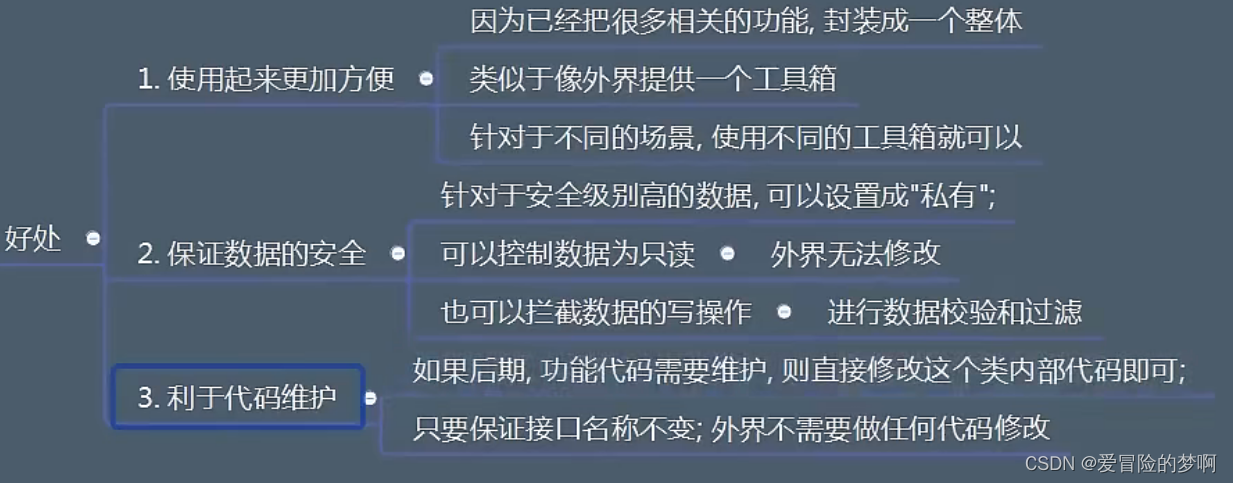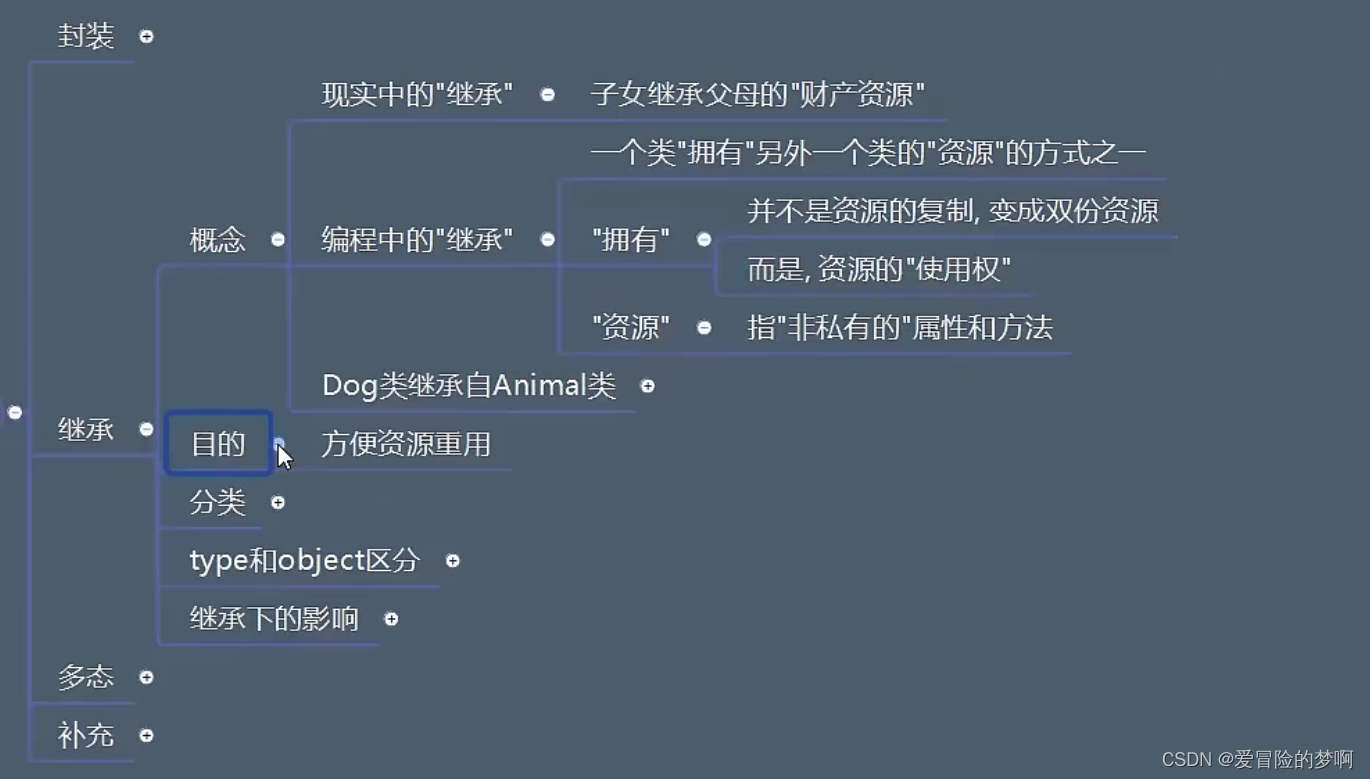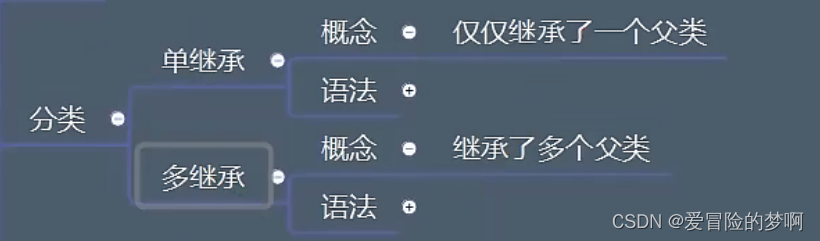``````class Animal():
pass
class Person():
pass
#单继承
class Dog(Animal):
pass

#多继承
class Monkey(Animal,Person):
pass
``````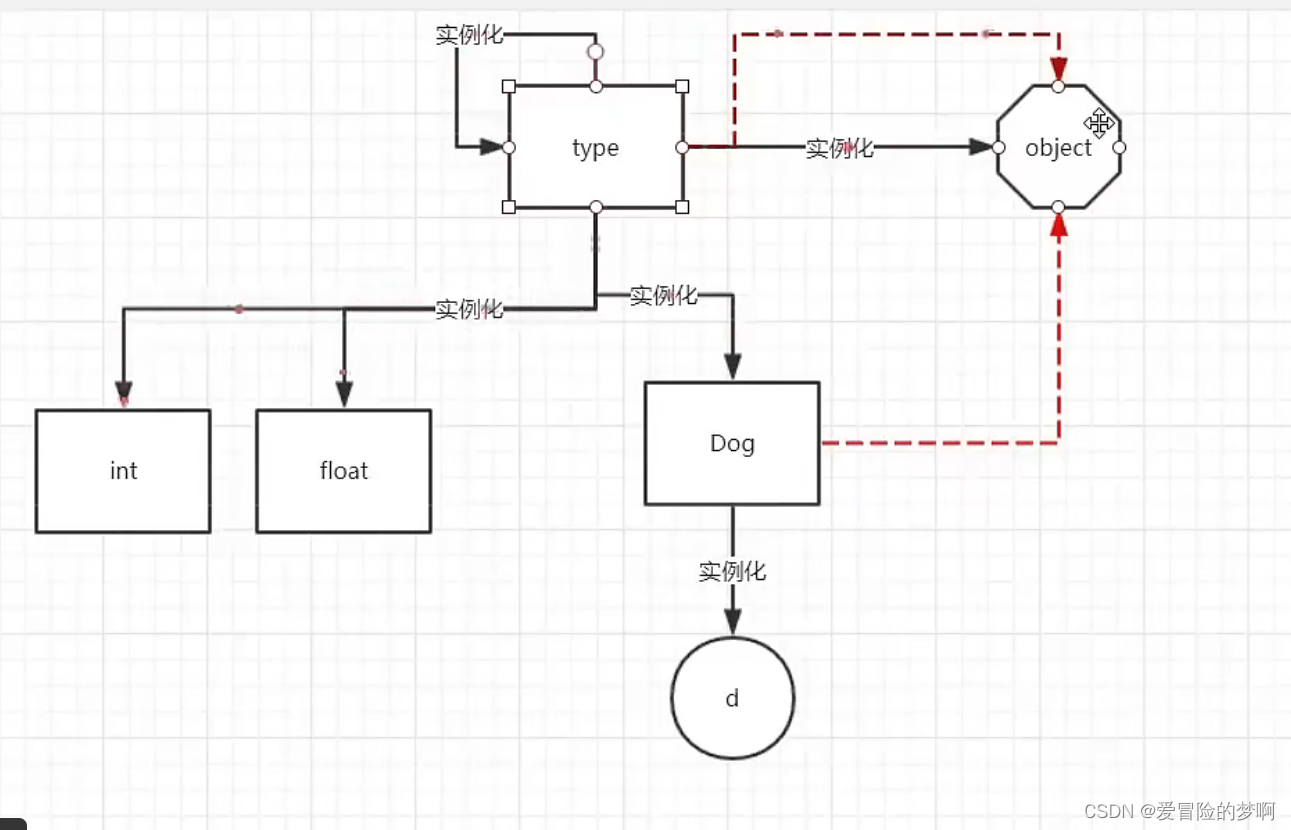• 继承的资源的使用：私有的属性和方法都无法访问，也就是带两个下划线的都不能访问；并且在python里面所谓的继承是指子类能不能使用父类的资源，并不是复制。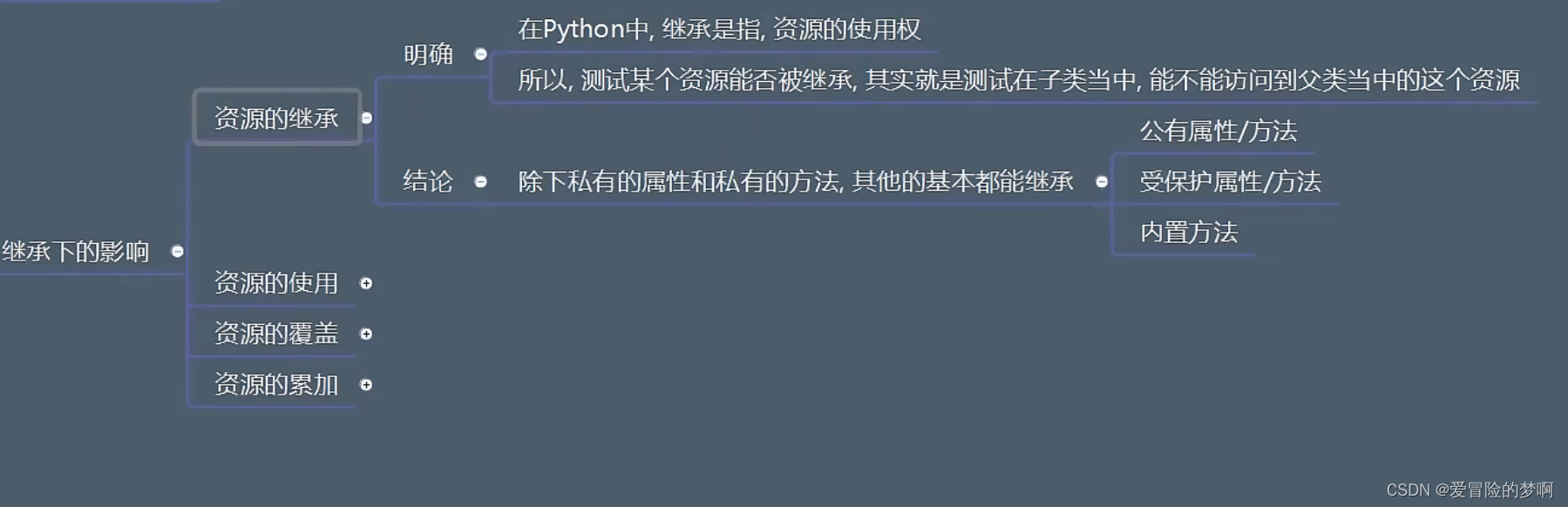``````
class A:
age = 9

class B(A):
pass

print(A.age)
print(B.age)
B.age = 10
print(A.age)
print(B.age)
print(A.__dict__)
print(B.__dict__)
``````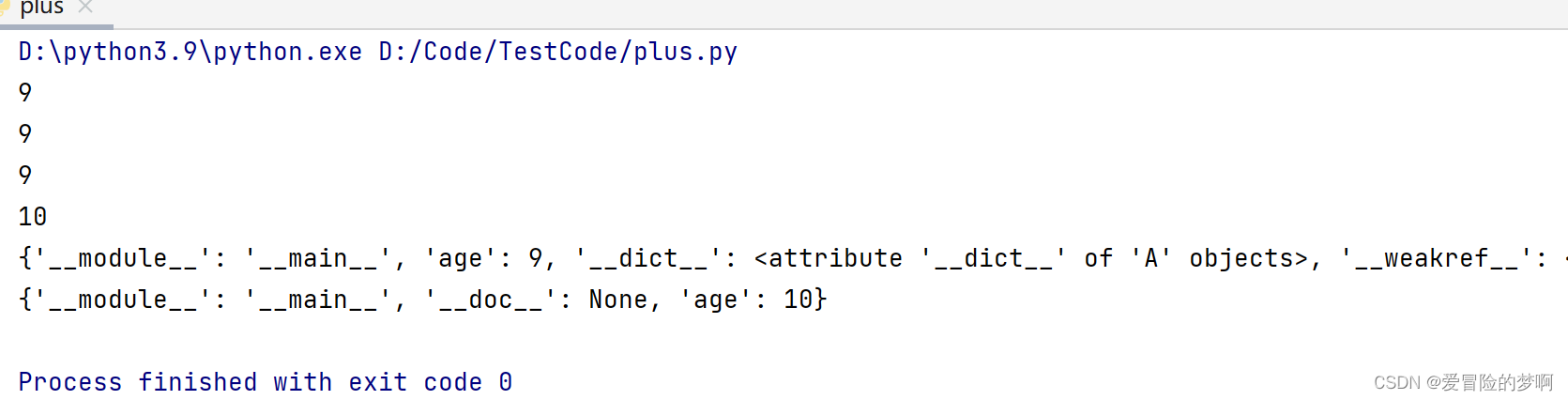• 常见的继承形态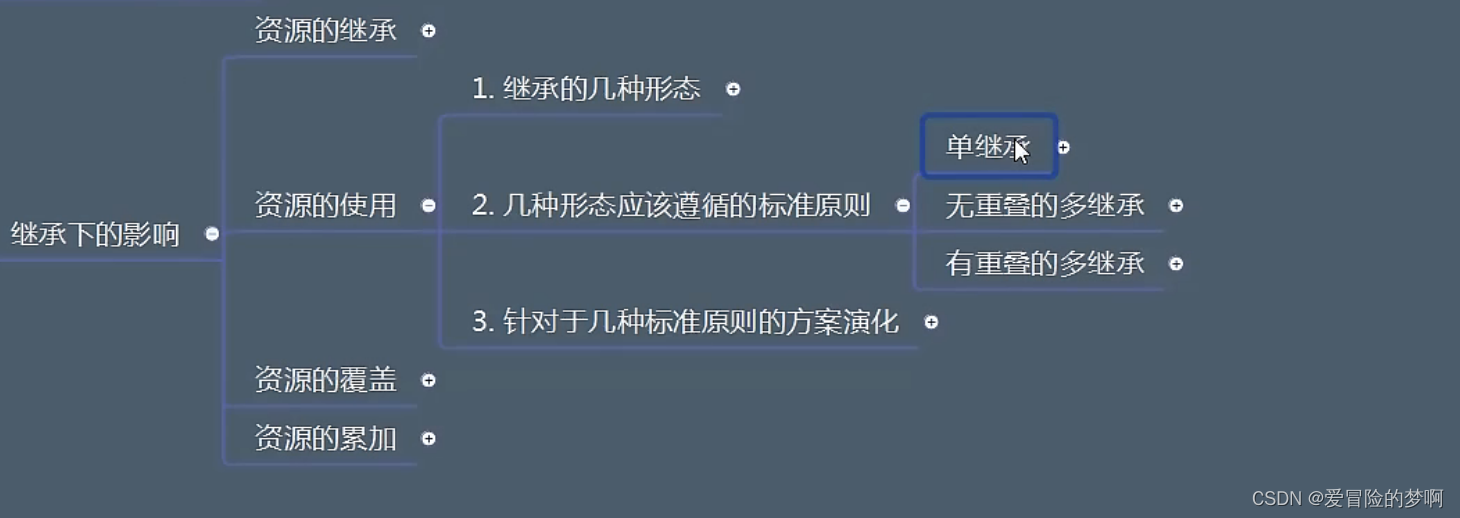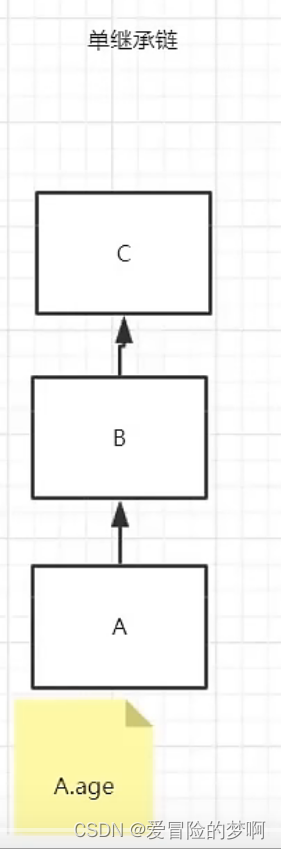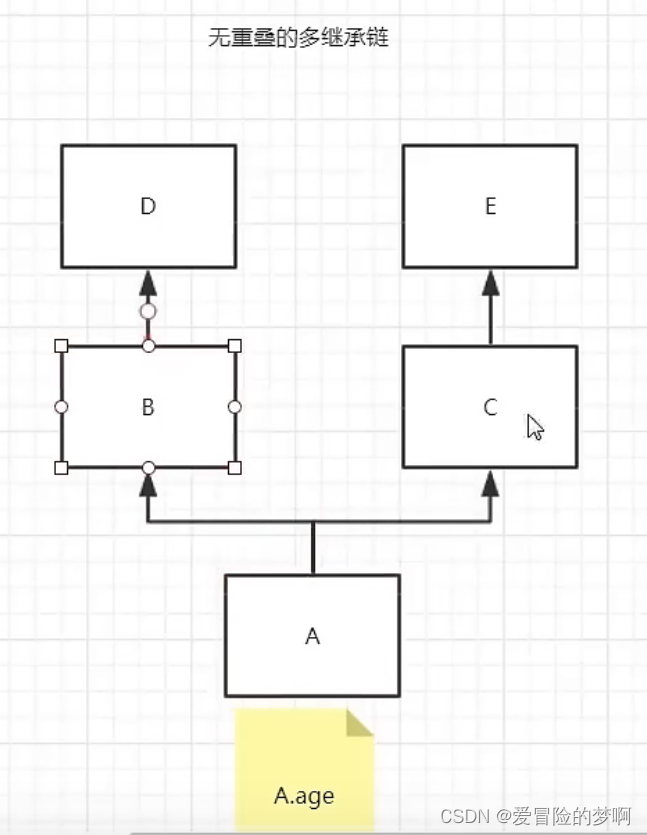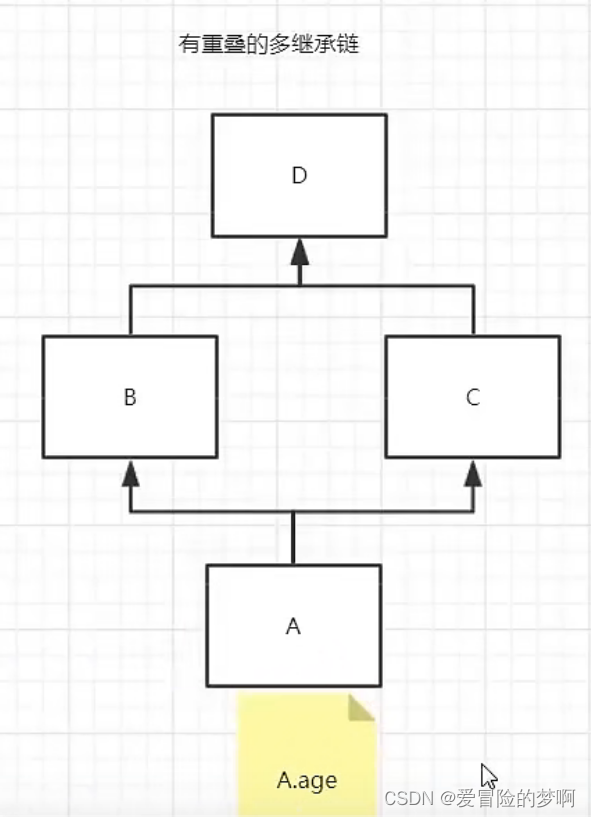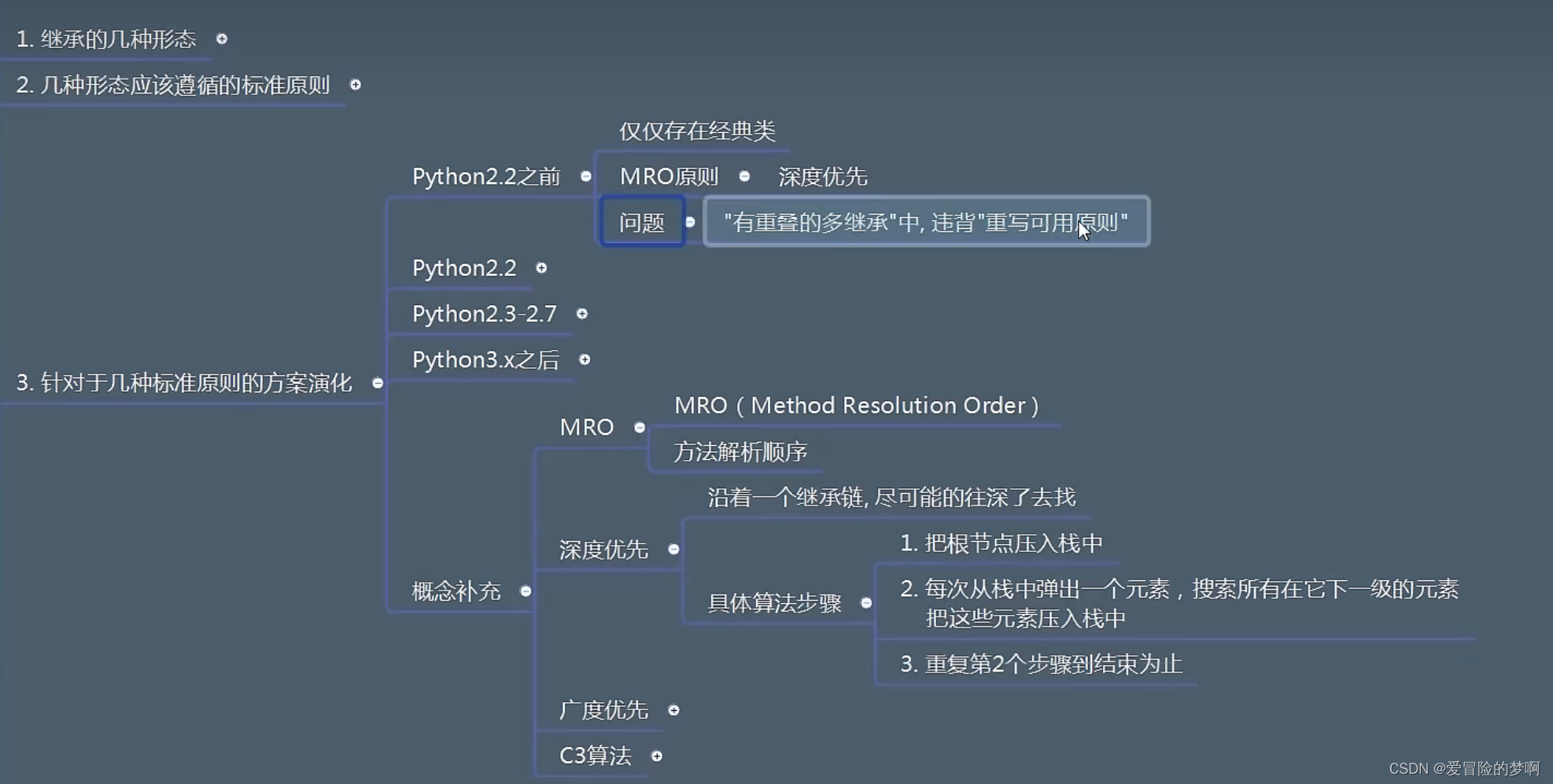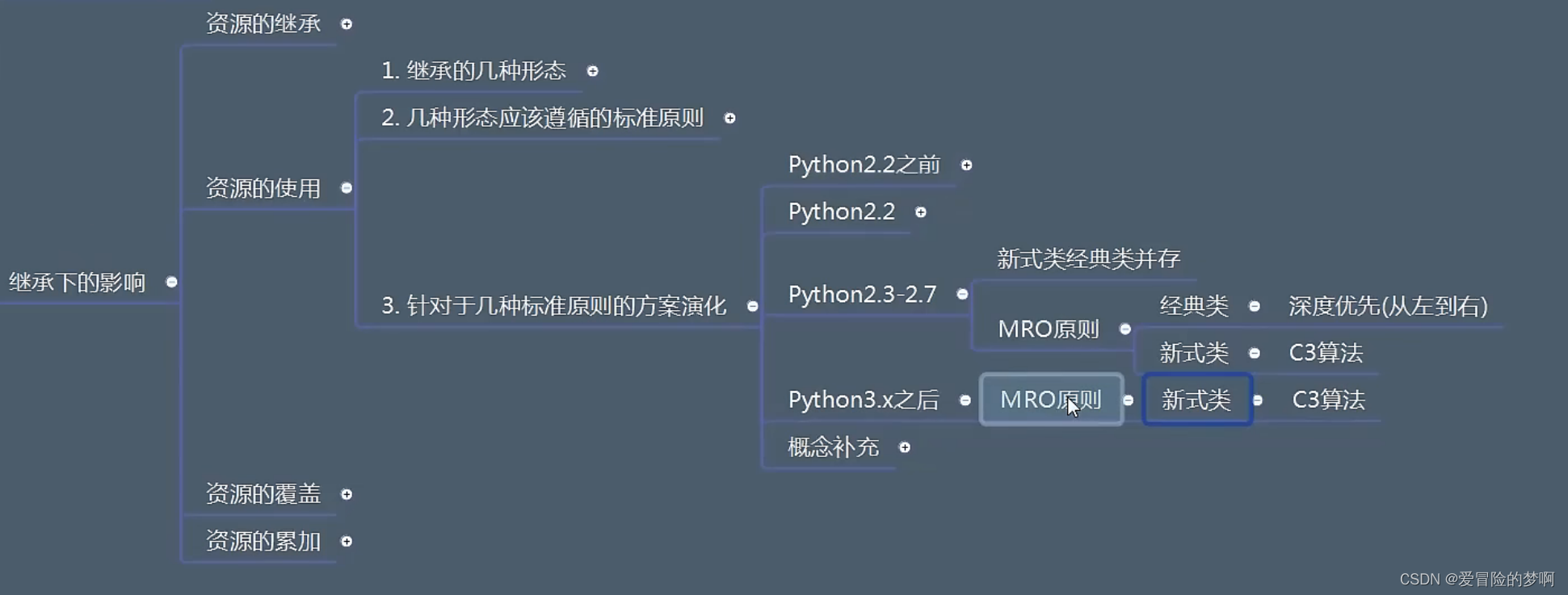• 资源的覆盖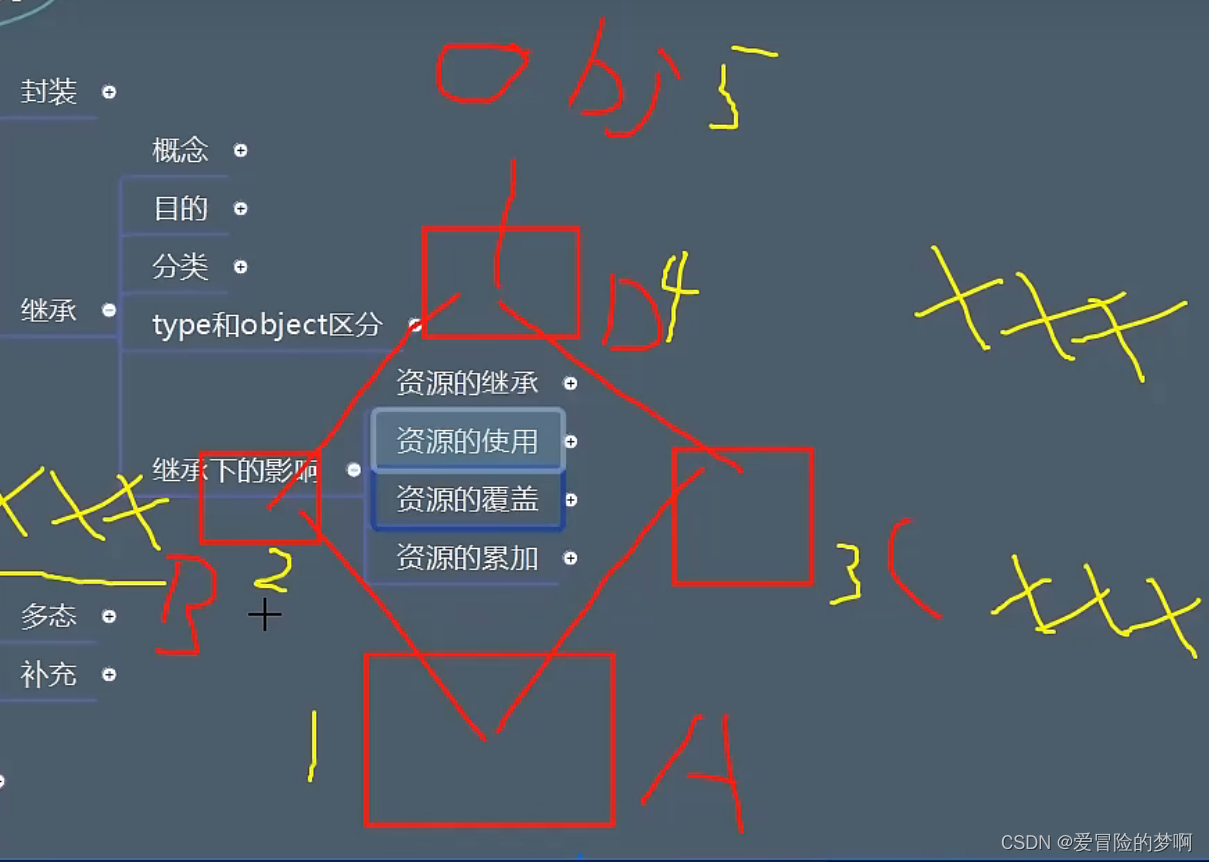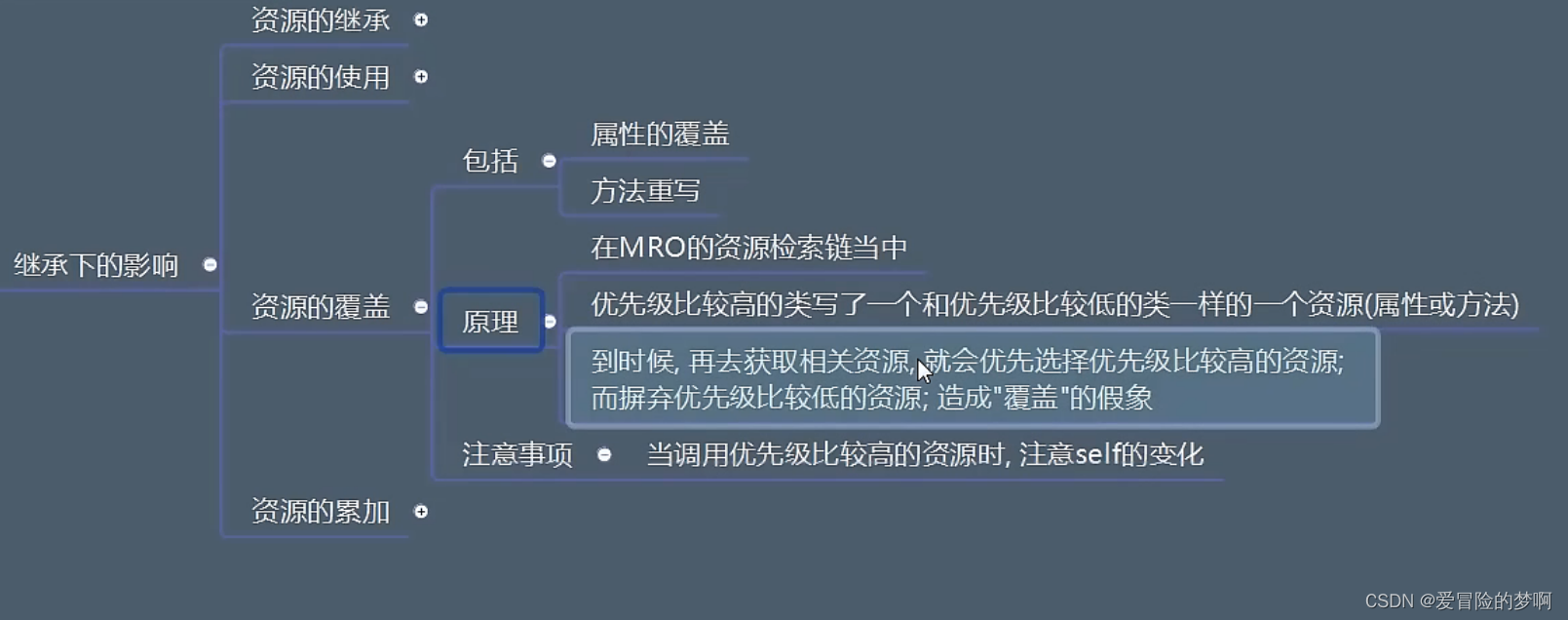• 资源的累加

``````class B:
age = 18
def __init__(self):
self.a = 1

class A(B):
def __init__(self):
self.b = 2

aa = A()
print(aa.b)
print(aa.a)
``````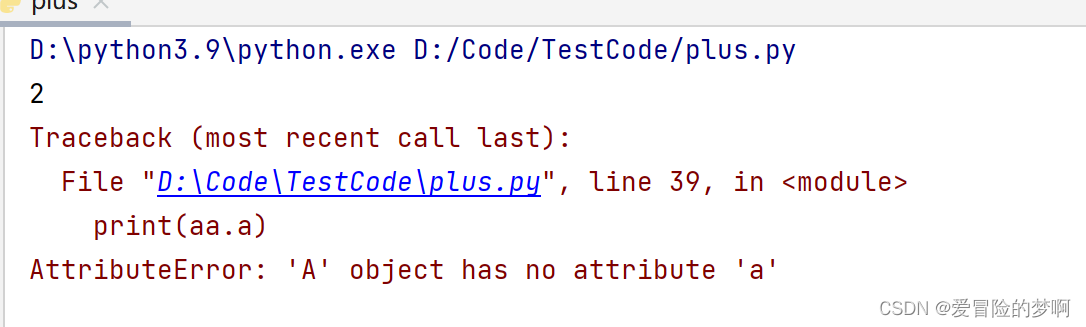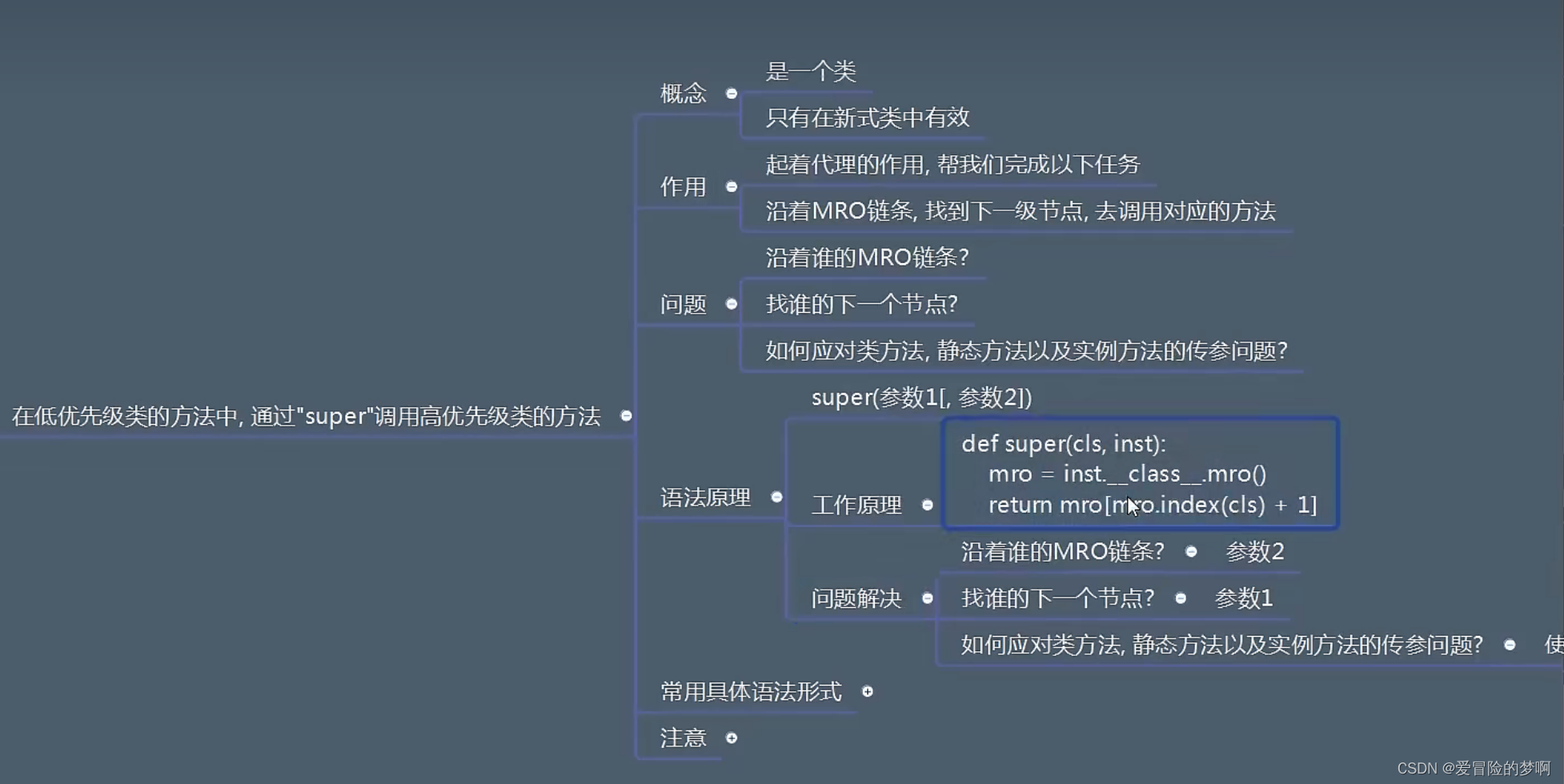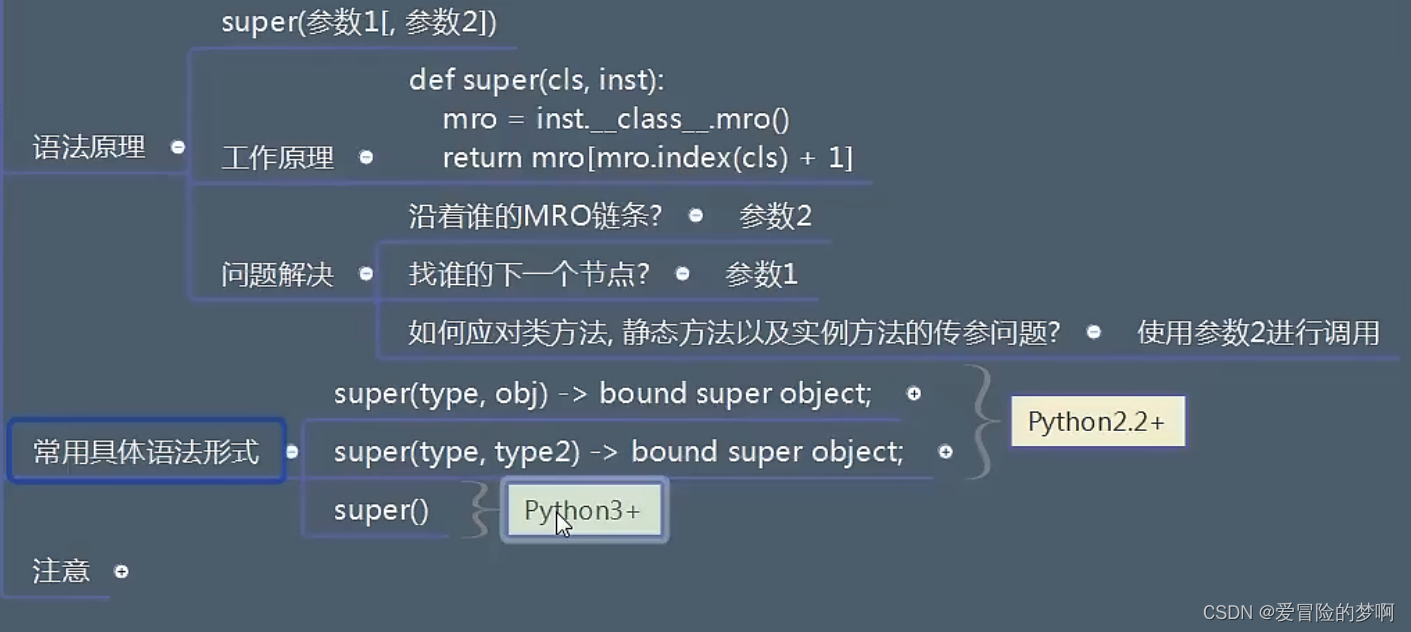``````class B:
a = 1

def __init__(self):
self.b = 2

def t1(self):
print('t1')

@classmethod
def t2(cls):
print('t2')

@staticmethod
def t3():
print('t3')

class A(B):
c = 3

def __init__(self):
# 这里我们看到要找A的下一个节点，第一个参数就传A
#对于第二个参数看沿着谁的链条，不能是B，因为B之后没有A了，只能是A（A的对象）或者A的子类（A子类对象）
#对于B的init这个方法，我们到时候要给这个实例方法里面传递参数self，是个实例对象；要是调用类方法传递的就是类了
#A的实例对象就是self
# super(A, self).__init__()
#上面的写法等同于下面，简化了会自动判断填充的
super().__init__()
self.e = 6

def tt1(self):
print('tt1')

@classmethod
def tt2(cls):
#这里因为是类方法（往B里面传的是类），所以第二个参数传入的是类，只能是A或者A的子类，这里只能写A
super(A,A).t2()
print('tt2')

@staticmethod
def tt3():
print('tt3')

a = A()
print(a.__dict__)
A.tt2()
``````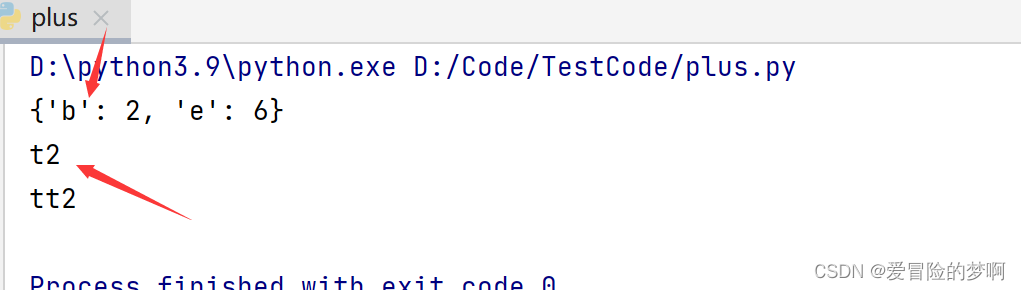``````class D():
def __init__(self):
print('d')

class B(D):
def __init__(self):
D.__init__(self)
print('b')

class C(D):
def __init__(self):
D.__init__(self)
print('c')

class A(B,C):
def __init__(self):
B.__init__(self)
C.__init__(self)
print('a')

A()
``````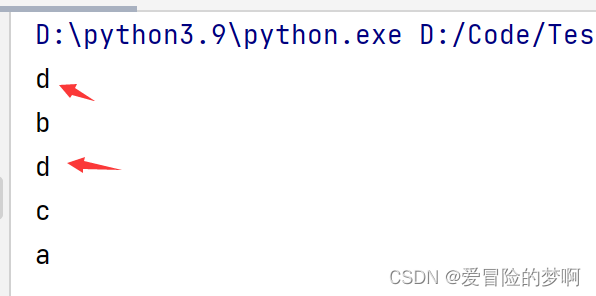``````class D():
def __init__(self):
print('d')

class B(D):
def __init__(self):
super().__init__()
print('b')

class C(D):
def __init__(self):
super().__init__()
print('c')

class A(B,C):
def __init__(self):
# B.__init__(self)
# C.__init__(self)
super().__init__()
print('a')

A()
``````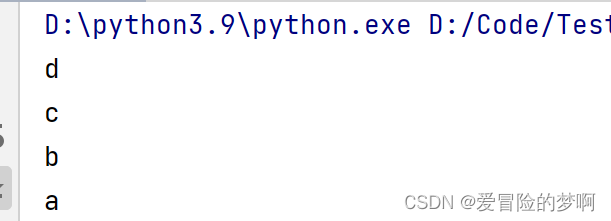``````
class Animal():
def jiao(self):
pass

class Dog(Animal):
def jiao(self):
print('汪汪汪')

class Cat(Animal):
def jiao(self):
print('喵喵喵')

def test(obj):
obj.jiao()

d = Dog()
c = Cat()
test(c)
``````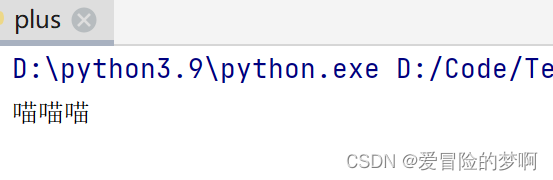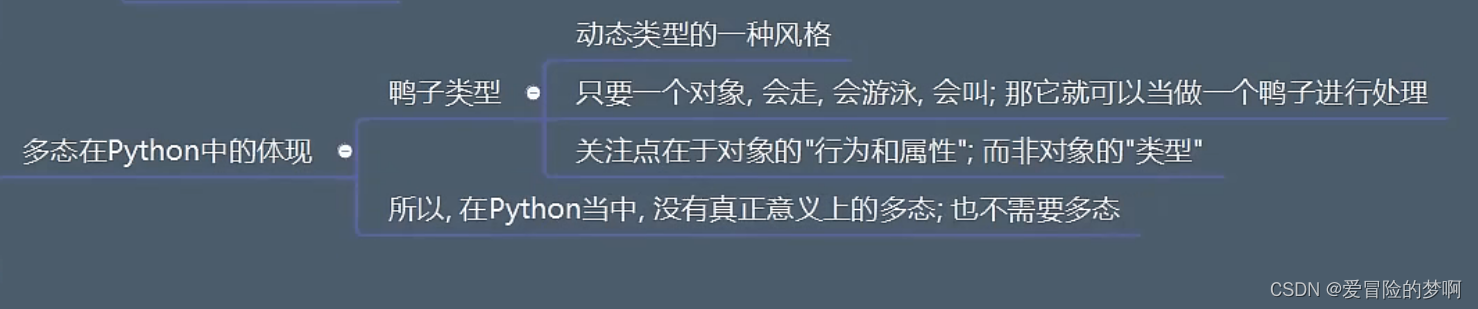• 抽象类和抽象方法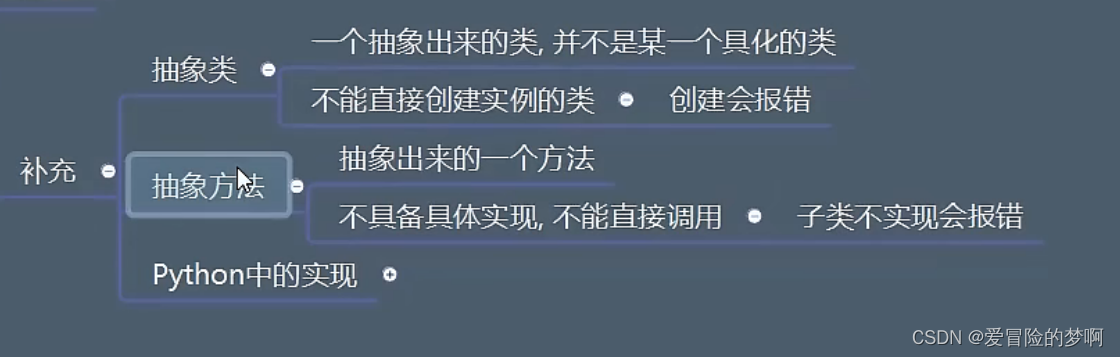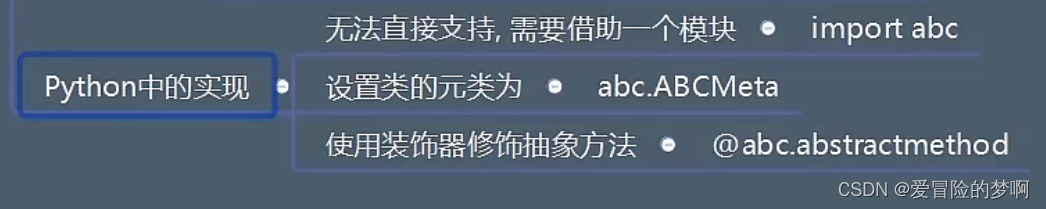``````import abc

class Animal(object,metaclass=abc.ABCMeta):
@abc.abstractmethod
def jiao(self):
pass
@abc.abstractclassmethod
def test(cls):
pass

class Dog(Animal):
def jiao(self):
print('汪汪汪')

@classmethod
def test(cls):
print('xxx')
class Cat(Animal):
def jiao(self):
print('喵喵喵')

def test(obj):
obj.jiao()

d = Dog()
d.jiao()
d.test()

``````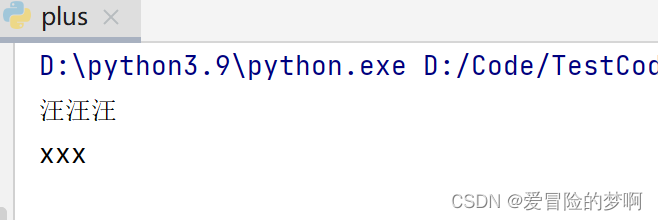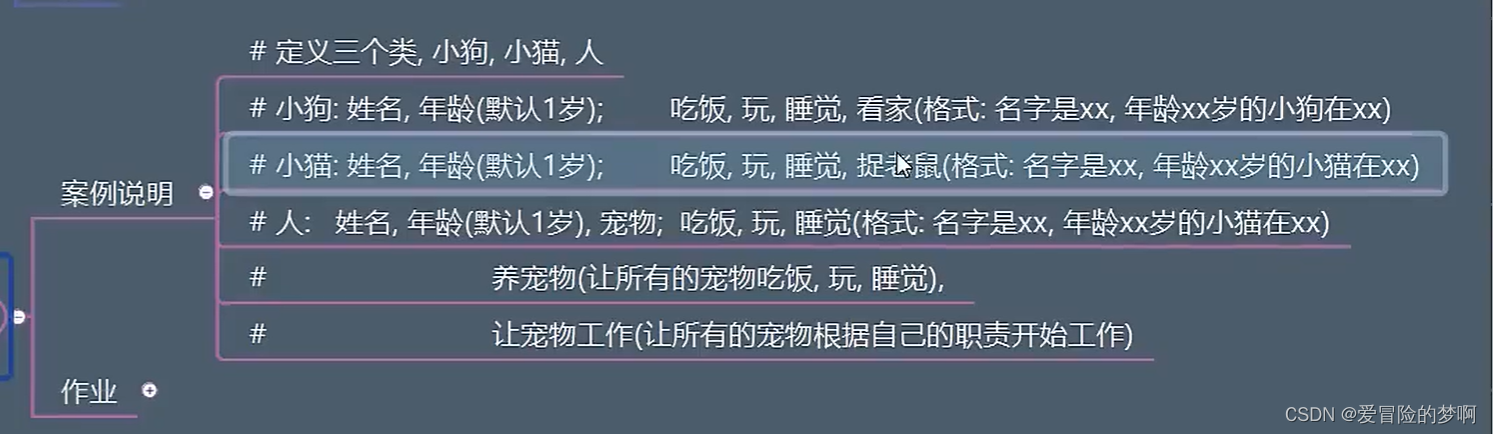``````class Dog:
#age设置为如果不写年龄默认为1
def __init__(self,name,age = 1):
self.name = name
self.age = age

def eat(self):
print(f'叫{self.name},{self.age}岁的小狗正在吃东西')

def play(self):
print(f'叫{self.name},{self.age}岁的小狗正在玩')

def sleep(self):
print(f'叫{self.name},{self.age}岁的小狗正在睡觉')

def watch(self):
print(f'叫{self.name},{self.age}岁的小狗正在看家')

d = Dog('小黑',10)
d.play()
``````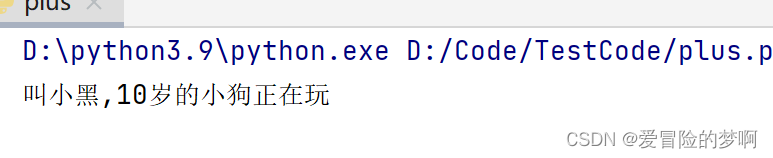• 改进1，我们可以看到之前写的print函数里面好多是重复的，所以我们可以选用之前学的str来提取公共的话语来简化，str表示self字符串本身它的字符串描述，到时候只需要把这个字符串返回出去即可
``````class Dog:
#age设置为如果不写年龄默认为1
def __init__(self,name,age = 1):
self.name = name
self.age = age

def eat(self):
print(f'{self}吃东西')

def play(self):
print(f'{self}玩')

def sleep(self):
print(f'{self}睡觉')

def watch(self):
print(f'{self}看家')

def __str__(self):
return f'叫{self.name}，{self.age}的小狗正在'
d = Dog('小黑',10)
d.play()
``````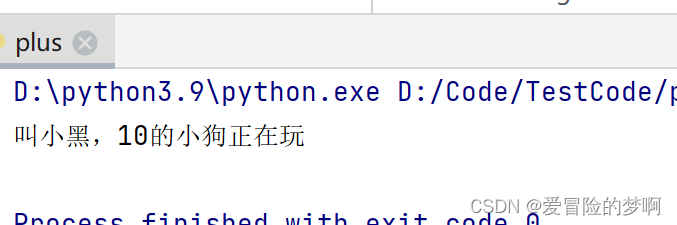• 改进二：加上猫和人这两个类别
``````class Person:
#这种存在默认值的放在最后面
def __init__(self,name,pets,age = 18):
self.name = name
self.age = age
self.pets = pets

def eat(self):
print(f'{self}吃东西')

def play(self):
print(f'{self}玩')

def sleep(self):
print(f'{self}睡觉')
#其实就是让所有的宠物吃饭，睡觉，玩
def carePets(self):
for pet in self.pets:
pet.eat()
pet.play()
pet.sleep()

#遍历拥有的宠物，根据宠物的类别不同让宠物进行各自的工作
def make_pets_work(self):
for pet in self.pets:
if isinstance(pet,Dog):
pet.watch()
elif isinstance(pet,Cat):
pet.catch()

def __str__(self):
return f'叫{self.name}，{self.age}的小狗正在'

class Cat:
#age设置为如果不写年龄默认为1
def __init__(self,name,age = 1):
self.name = name
self.age = age

def eat(self):
print(f'{self}吃东西')

def play(self):
print(f'{self}玩')

def sleep(self):
print(f'{self}睡觉')

def catch(self):
print(f'{self}捉老鼠')

def __str__(self):
return f'叫{self.name}，{self.age}的小猫正在'

class Dog:
#age设置为如果不写年龄默认为1
def __init__(self,name,age = 1):
self.name = name
self.age = age

def eat(self):
print(f'{self}吃东西')

def play(self):
print(f'{self}玩')

def sleep(self):
print(f'{self}睡觉')

def watch(self):
print(f'{self}看家')

def __str__(self):
return f'叫{self.name}，{self.age}的小狗正在'

d = Dog('小黑',10)
c = Cat('小红',6)
p = Person('sz',[d,c],18)
p.carePets()
p.make_pets_work()
``````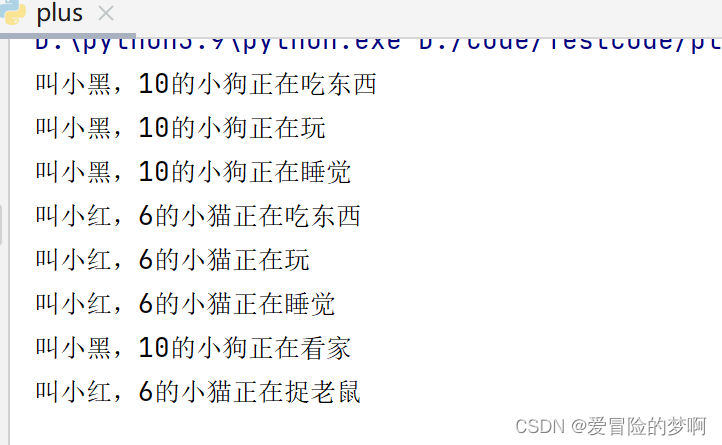• 改进三：我们知道如果一个人拥有很多类别的宠物的时候，这个代码就要继续加判断，很麻烦，所以我们在宠物的方法中统一成work方法，这样就不用判断了
• 改进四：每个类有很多是相同的，所以我们可以使用三大特性之继承特性，写一个父类，把公共方法什么的写到父类里面去，然后用别的去继承，再加上各自的单独的属性即可

``````class Animial():
def __init__(self, name, age=18):
self.name = name
self.age = age

def eat(self):
print(f'{self}吃东西')

def play(self):
print(f'{self}玩')

def sleep(self):
print(f'{self}睡觉')

class Person(Animial):
#加super是继承父类的属性
def __init__(self,name,pets,age = 18):
super(Person,self).__init__(name,age)
self.pets = pets

def carePets(self):
for pet in self.pets:
pet.eat()
pet.play()
pet.sleep()

#遍历拥有的宠物，根据宠物的类别不同让宠物进行各自的工作
def make_pets_work(self):
for pet in self.pets:
pet.work()

def __str__(self):
return f'叫{self.name}，{self.age}的小狗正在'

class Cat(Animial):

def work(self):
print(f'{self}捉老鼠')

def __str__(self):
return f'叫{self.name}，{self.age}的小猫正在'

class Dog(Animial):

def work(self):
print(f'{self}看家')

def __str__(self):
return f'叫{self.name}，{self.age}的小狗正在'

d = Dog('小黑',10)
c = Cat('小红',6)
p = Person('sz',[d,c],18)
p.carePets()
p.make_pets_work()
``````